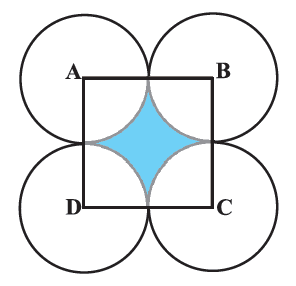# Ex.12.3 Q7 Areas Related to Circles Solution - NCERT Maths Class 10

Go back to  'Ex.12.3'

## Question

In the given figure, $$ABCD$$ is a square of side $$\text{14 cm.}$$ With Centers $$A, B, C$$ and $$D,$$ four circles are drawn such that each circle touch externally two of the remaining three circles. Find the area of the shaded region.Video Solution
Areas Related To Circles
Ex 12.3 | Question 7

## Text Solution

What is known?

$$ABCD$$ is a square of side $$\text{= 14 cm.}$$

With centers$$A, B, C, D$$ four circles are drawn such that each circle touches externally $$2$$ of the remaining $$3$$ circles.

What is unknown?

Reasoning:

Since the circles are touching each other externally,visually it is clear that

Radius of each circle \begin{align} {r} = \frac{1}{2}\,\, \times \end{align}(side of square)

Also, $$ABCD$$ being a square all angles are of measure $${90^ \circ },\,\,\,$$

Therefore,all sector are equal as they have same radii and angle.

$$\therefore\;$$ Angle of each sector which is part of the square $$\left( {\theta } \right) = {90^ \circ }$$

$$\therefore\;$$$$\text{Area of each sector}$$\begin{align}&= \frac{\theta }{{{{360}^\circ }}} \times \pi {r^2}\\&= \frac{{{{90}^\circ }}}{{{{360}^\circ }}} \times \pi {r^2}\\&= \frac{{\pi {r^2}}}{4}\end{align}

From the figure it is clear that:

Area of shaded region $$=$$  Area of square $$–$$  Area of $$4$$ sectors

\begin{align} &= {\left( {{\text{side}}} \right)^2} - 4 \times {\text{Area of each sector}}\\ &= {\left( {14} \right)^2} - 4 \times \frac{{\pi {r^2}}}{4}\\ &= {\left( {14} \right)^2} - \pi {r^2}\end{align}

Steps:

Area of each of the $$4$$ sectors is equal as each sector subtends an angle of $${90^ \circ }$$ at the centre of a circle with radius $$\text{= 7 cm}$$

$$\therefore$$ $$\text{Area of each sector}$$\begin{align}&= \frac{\theta }{{{{360}^\circ }}} \times \pi {r^2}\\ &= \frac{{{{90}^\circ }}}{{{{360}^\circ }}} \times \pi {(7)^2}\\ &= \frac{1}{4} \times \frac{{22}}{7} \times 7 \times 7\\ &= \frac{{77}}{2}{\text{c}}{{\text{m}}^2}\end{align}

Area of shaded region $$=$$  Area of square $$- \,4 \;\times$$ Area of each sector

\begin{align}&= {(14)^2} - 4 \times \frac{{77}}{2}\\ &= 196 - 154\\ &= 42\,\,{\text{c}}{{\text{m}}^2}\end{align}

Learn from the best math teachers and top your exams

• Live one on one classroom and doubt clearing
• Practice worksheets in and after class for conceptual clarity
• Personalized curriculum to keep up with school